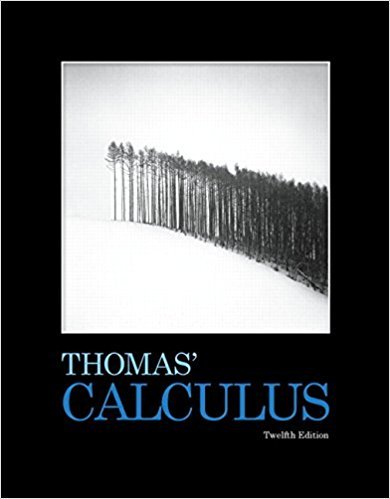×
×

# Solutions for Chapter 9: First-Order Differential Equations## Full solutions for Thomas' Calculus | 12th Edition

ISBN: 9780321587992Solutions for Chapter 9: First-Order Differential Equations

Solutions for Chapter 9
4 5 0 324 Reviews
13
0
##### ISBN: 9780321587992

This expansive textbook survival guide covers the following chapters and their solutions. Chapter 9: First-Order Differential Equations includes 36 full step-by-step solutions. Thomas' Calculus was written by and is associated to the ISBN: 9780321587992. Since 36 problems in chapter 9: First-Order Differential Equations have been answered, more than 8190 students have viewed full step-by-step solutions from this chapter. This textbook survival guide was created for the textbook: Thomas' Calculus, edition: 12.

Key Calculus Terms and definitions covered in this textbook
• Central angle

An angle whose vertex is the center of a circle

• Components of a vector

See Component form of a vector.

• Divisor of a polynomial

See Division algorithm for polynomials.

• Double-blind experiment

A blind experiment in which the researcher gathering data from the subjects is not told which subjects have received which treatment

• Factored form

The left side of u(v + w) = uv + uw.

• Five-number summary

The minimum, first quartile, median, third quartile, and maximum of a data set.

• Index

• Infinite discontinuity at x = a

limx:a + x a ƒ(x) = q6 or limx:a - ƒ(x) = q.

• Inverse of a matrix

The inverse of a square matrix A, if it exists, is a matrix B, such that AB = BA = I , where I is an identity matrix.

• Inverse relation (of the relation R)

A relation that consists of all ordered pairs b, a for which a, b belongs to R.

• Main diagonal

The diagonal from the top left to the bottom right of a square matrix

• Numerical derivative of ƒ at a

NDER f(a) = ƒ1a + 0.0012 - ƒ1a - 0.00120.002

• Parametric curve

The graph of parametric equations.

• Product rule of logarithms

ogb 1RS2 = logb R + logb S, R > 0, S > 0,

• Quotient identities

tan ?= sin ?cos ?and cot ?= cos ? sin ?

• Root of an equation

A solution.

• Slant asymptote

An end behavior asymptote that is a slant line

• Spiral of Archimedes

The graph of the polar curve.

• Terms of a sequence

The range elements of a sequence.

• Vertical stretch or shrink

See Stretch, Shrink.

×